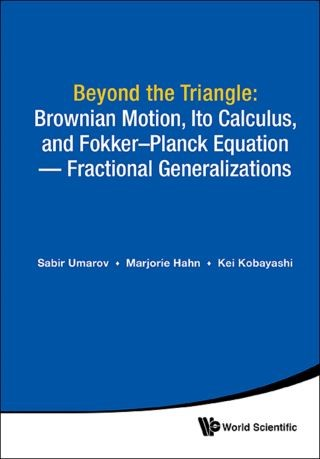## Beyond the Triangle• Description
• Author
• Info
• Reviews

### Description

The book is devoted to the fundamental relationship between three objects: a stochastic process, stochastic differential equations driven by that process and their associated Fokker–Planck–Kolmogorov equations. This book discusses wide fractional generalizations of this fundamental triple relationship, where the driving process represents a time-changed stochastic process; the Fokker–Planck–Kolmogorov equation involves time-fractional order derivatives and spatial pseudo-differential operators; and the associated stochastic differential equation describes the stochastic behavior of the solution process. It contains recent results obtained in this direction.

This book is important since the latest developments in the field, including the role of driving processes and their scaling limits, the forms of corresponding stochastic differential equations, and associated FPK equations, are systematically presented. Examples and important applications to various scientific, engineering, and economics problems make the book attractive for all interested researchers, educators, and graduate students.

Contents:
• Introduction
• The Original Triangle: Brownian Motion, Itô Stochastic Calculus, and Fokker–Planck–Kolmogorov Equation
• Fractional Calculus
• Pseudo–Differential Operators Associated with Lévy Processes
• Stochastic Processes and Time-Changes
• Stochastic Calculus for Time-Changed Semimartingales and Its Applications to SDEs
• Fractional Fokker–Planck–Kolmogorov Equations